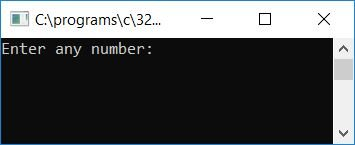Perfect Number in C Program

# Perfect Number in C Program

## heck Perfect Number or Not in C

The question is, write a program in C that checks whether a given number is a perfect number or not. The program given below is its answer. 6 is a perfect number because 1, 2, and 3 are the three factors of 6 and after summing it up, you will get the same number itself as in case of 6:

```#include<stdio.h>
#include<conio.h>
int main()
{
int num, sum=0, i;
printf("Enter any number: ");
scanf("%d", &num);
for(i=1; i<num; i++)
{
if(num%i == 0)
sum = sum + i;
}
if(num == sum)
printf("\nIt's a Perfect Number.");
else
printf("\nIt's not a Perfect Number.");
getch();
return 0;
}```

The above program was build and run under Code::Blocks IDE, here is the output. This is first snapshot of the sample run:Supply any number say 6 and press `ENTER` key to see that the given number is a perfect number or not. This is the second snapshot of the sample run: